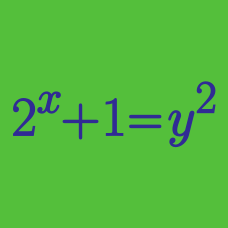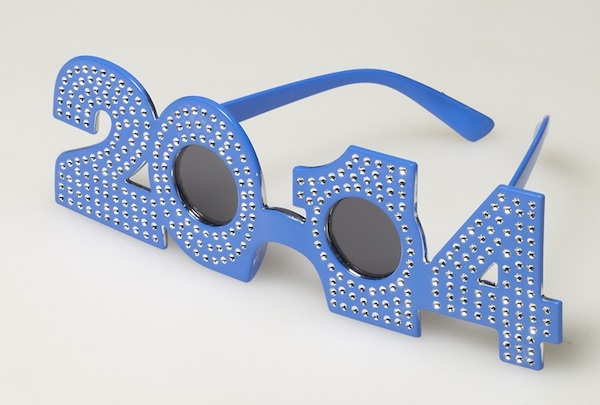Algebra

# Algebraic Manipulation: Level 2 Challenges

If $a-b=4$ and $ab=45$, what is the value of $a^3 -b^3$?

Given that $a$, $b$ and $c$ are real numbers such that $abc=1$, find the value of $\left(\frac{a}{ab+a+1}+\frac{b}{bc+b+1}+\frac{c}{ca+c+1}\right)^2.$

$\large \frac{1 + 3 + 5 + 7 +\ldots+ 199}{2 + 4 + 6 + 8 +\ldots+ 200} = \ ?$$\large \frac{1^2+2^2+3^2+\cdots+2012^2+2013^2+2014^2}{1+2+3+\cdots+2012+2013+2014} = \ ?$

Hint: $1^2 + 2^2 + 3^2 + \cdots + n^2= \frac16 n(n+1)(2n+1)$.

$\large { \begin{cases} {x^2-y^2=4-2xy} \\ { x+ y = 2 } \end{cases} }$

If $x$ and $y$ satisfy the system of equations above, find the value of $x-y$.

×

Problem Loading...

Note Loading...

Set Loading...